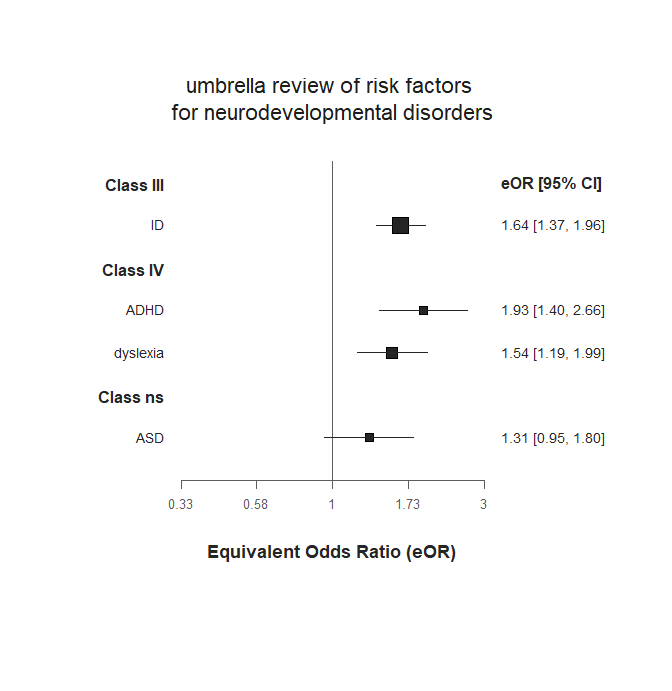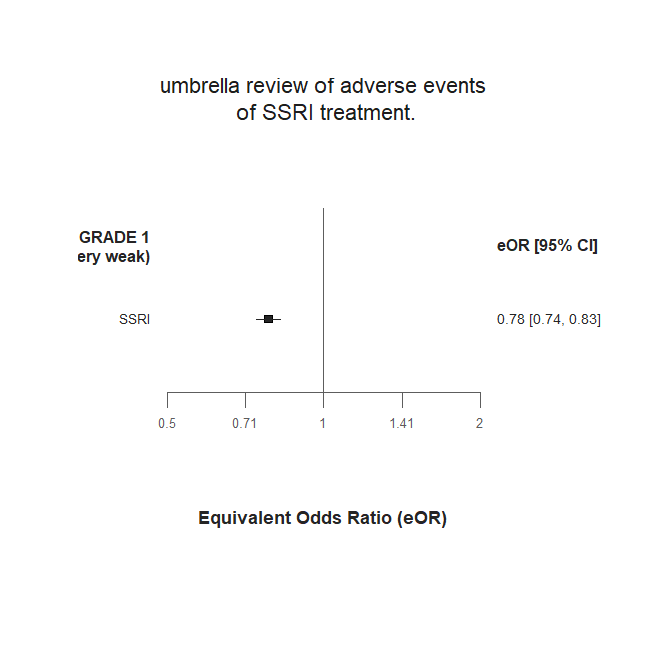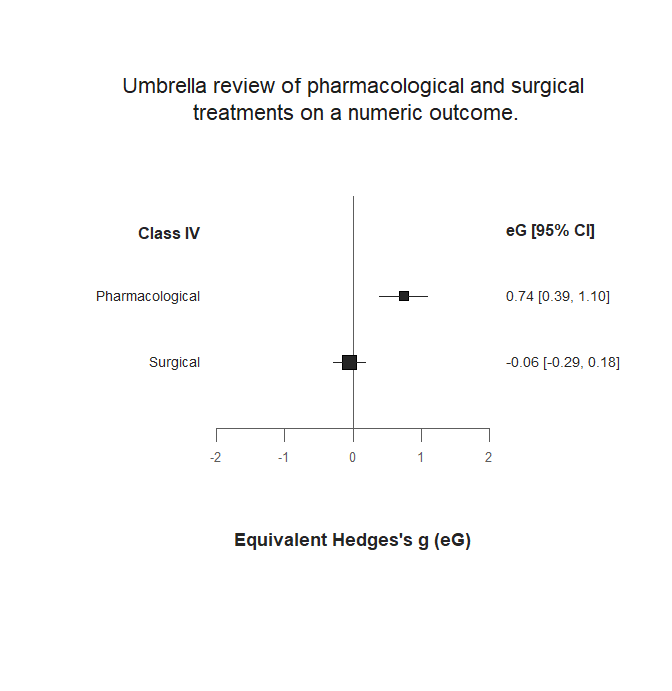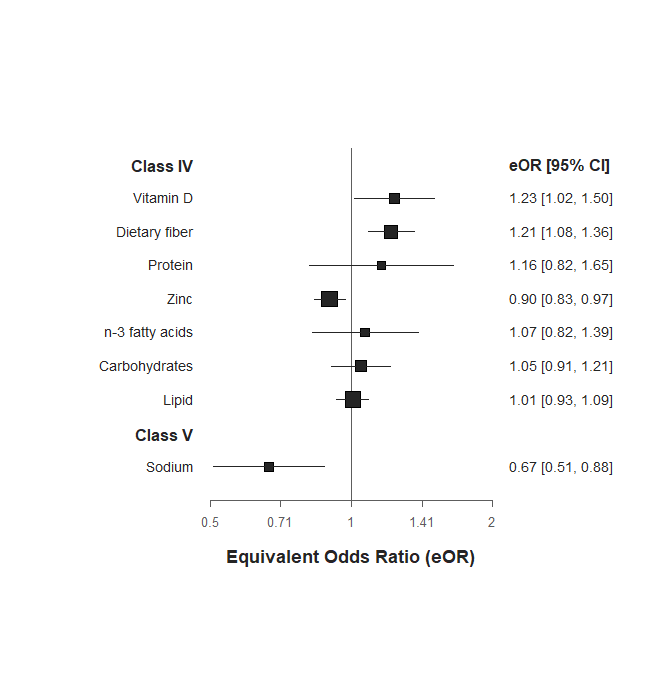# Introduction

The metaumbrella package offers several facilities to assist in data analysis when performing an umbrella review. More precisely, this package is built around three core functions which aim to facilitate (i) the completion of the statistical analyses required for an umbrella review, (ii) the stratification of the evidence and (iii) the graphical presentation of the results of an umbrella review.

In this document, we present a very brief description of the core functions available in the metaumbrella package. Then, we give several concrete examples of umbrella reviews conducted in R using this package.

# Description of the metaumbrella package

The package includes 3 core functions:

1. the umbrella() function
2. the add.evidence() function
3. the forest() function

1. umbrella() The umbrella() function allows to perform the calculations required to stratify the evidence. The main argument required by this function is a well-formatted dataset. How properly formatting your dataset is beyond the scope of this vignette but general guidance can be found in the manual of this package and another vignette is specifically dedicated to this issue.
Once your dataset has been correctly formatted, it is used as an argument of the umbrella function, which automatically:

• performs random-effects meta-analyses.
• provides an estimation of the between-study variance and heterogeneity using three indicators (tau2, Q-statistic and I2 statistic).
• estimates the 95% prediction interval.
• estimates the statistical significance of the largest study included in each meta-analysis.
• assesses publication bias using the Egger’s test.
• assesses excess of significance bias
• performs a jackknife leave-one-out meta-analysis.
• calculates the proportion of participants included in studies at low risk of bias (if study quality is indicated in the dataset).

For example, using df.SMD as a well-formatted dataset, you can automatically perform all the calculations required for an umbrella review using this R code:

umb <- umbrella(df.SMD)

2. add.evidence() The add.evidence() function uses the calculations performed by the umbrella() function to perform a stratification of evidence according to algorithmic criteria:

• Ioannidis: this criteria strictly applies the classification described in Fusar-Poli & Radua (2018).
• GRADE: this criteria proposes to stratify the evidence according to algorithmic criteria inspired from the GRADE.
• Personalized: this criteria allows users to chose up to 13 criteria to stratify the evidence.

Ioannidis criteria

To obtain a stratification of evidence according to the Ioannidis criteria, it only requires to specify criteria = "Ioannidis" in the add.evidence() function.

umb <- umbrella(df.SMD)
strat.io <- add.evidence(umb.SMD, criteria = "Ioannidis")

To obtain a stratification of evidence according to the GRADE criteria, it only requires to specify criteria = "GRADE" in the add.evidence() function.

umb <- umbrella(df.SMD)
strat.grd <- add.evidence(umb.SMD, criteria = "GRADE")

Personalized criteria

Up to 13 criteria can be used to stratify evidence in this Personalized classification.

• n_studies: a number of studies included in the meta-analysis. If the number of studies included in the meta-analysis is strictly superior to the threshold value indicated in this n_studies criteria, the class for which this value is indicated can be reached.
• total_n: a total number of participants included in the meta-analysis. If the total number of participants included in the meta-analysis is strictly superior to the threshold value indicated in this total_n criteria, the class for which this value is indicated can be reached.
• n_cases: a number of cases included in the meta-analysis. If the number of cases included in the meta-analysis is strictly superior to the threshold value indicated in this n_cases criteria, the class for which this value is indicated can be reached.
• p_value: a p-value of the pooled effect size under the random-effects model. If the p-value of the pooled effect size is strictly inferior to the threshold value indicated in this p_value criteria, the class for which this value is indicated can be reached.
• I2: an I value. If the I value of the meta-analysis is strictly inferior to the threshold value indicated in this I2 criteria, the class for which this value is indicated can be reached.
• imprecision: an SMD value that will be used to calculate the statistical power of the meta-analysis. If the number of participants included in the meta-analyses allows obtaining a statistical power strictly superior to 80% for the SMD value indicated in this imprecision criteria, the class for which this value is indicated can be reached.
• rob: a percentage of participants included in studies at low risk of bias. Note that that the approach to determining whether a study is at low risk of bias is left to the user. If the percentage of participants included in studies at low risk of bias is strictly superior to the threshold value indicated in this rob criteria, the class for which this value is indicated can be reached.
• amstar: an AMSTAR rating on the methodological quality of the meta-analysis. If the AMSTAR value of the meta-analysis is strictly superior to the threshold value indicated in this amstar criteria, the class for which this value is indicated can be reached.
• egger_p: a p-value of an Egger’s test for publication bias. If the p-value of the Egger’s test is strictly superior to the threshold value indicated in this egger_p criteria, the class for which this value is indicated can be reached.
• esb_p: a p-value of a test for excess of significance bias (ESB). If the p-value of the test is strictly superior to the threshold value indicated in this esb_p criteria, the class for which this value is indicated can be reached.
• JK_p: the largest p-value obtained in the jackknife meta-analysis (JK). If the largest p-value obtained in the jackknife meta-analysis is strictly lower to the threshold value indicated in JK_p, the class for which this value is indicated can be reached.
• pi: a “notnull” value indicates that the user requests the 95% prediction interval of the meta-analysis to exclude the null value to achieve the class for which it is indicated.
• largest_CI: a “notnull” value indicates that the user requests the 95% confidence interval of the largest study included in the meta-analysis to exclude the null value to achieve the class for which it is indicated.

In contrast to the two previous classifications, the Personalized criteria requires to manually indicate several cut-off values for each criteria you plan to use. Examples of stratification of the evidence according to the ‘Personalized’ classification can be found in the Example 3 and Example 4 of this vignette.

A brief example of stratification of the evidence according to the Personalized classification can be:

umb <- umbrella(df.SMD)
strat.prso <- add.evidence(umb, criteria = "Personalized",
class_I = c(total_n = 600, I2 = 25, rob = 75),
class_II = c(total_n = 400, I2 = 50, rob = 50),
class_III = c(total_n = 200, I2 = 75, rob = 25),
class_IV = c(total_n = 100))

3. forest() The forest() function allows to have a visualization of the results of the umbrella review

umb <- umbrella(df.SMD)
strat.io <- add.evidence(umb.SMD, criteria = "Ioannidis")
forest(strat.io)

# Example 1: “Ioannidis” classification

This example uses the dataset named df.OR distributed along with the metaumbrella package. You can access and visualize the dataset in R with the following command

df.OR

Because the dataset includes four factors (ASD, ADHD, ID and dyslexia), the calculations and the stratification of evidence will be performed independently for each of these factors.

To perform the calculations, simply apply the umbrella function on this well-formatted dataset.

umb.OR <- umbrella(df.OR)
summary(umb.OR)

This output shows the results of the calculations conducted by the umbrella() function. Results are presented independently for each factor included in the dataset.

Once calculations have been performed via the umbrella function, you can stratify the evidence with the add.evidence() function. Here, we present an example of stratification according to the “Ioannidis” criteria.

strat.io <- add.evidence(umb.OR, criteria = "Ioannidis")
summary(strat.io)

A visual description of the results can be obtained using the forest() function. More information on how generating nice plots using the forest() function can be found in another vignette dedicated to this function.

forest(strat.io,
measure = "eOR",
main_title = "umbrella review of risk factors \nfor neurodevelopmental disorders")This example uses the dataset named df.RR distributed along with the metaumbrella package. You can access and visualize the dataset in R with the following command

df.RR

The dataset includes only one factor. To perform the calculations required for the stratification of evidence, simply apply the umbrella function on the df.RR well-formatted dataset.

umb.RR <- umbrella(df.RR)
summary(umb.RR)

This output shows the results of the calculations for the factor included in the dataset.

Once the calculations have been performed via the umbrella function, you can stratify the evidence with the add.evidence() function. Here, we present an example of stratification according to the “GRADE” criteria.

strat.grade <- add.evidence(umb.RR, criteria = "GRADE")
summary(strat.grade)

A visual description of the results can be obtained using the forest() function. More information on how generating nice plots using the forest() function can be found in another vignette dedicated to this function.

forest(strat.grade,
measure = "eOR",
main_title = "umbrella review of adverse events\n of SSRI treatment.")# Example 3: “Personalized” classification

This example uses the dataset named df.SMD distributed along with the metaumbrella package. You can access and visualize the dataset in R with the following command

df.SMD

Because the dataset includes two factors, the umbrella review will consider these factors as independent. The calculations and the stratification of evidence will be performed independently for these two factors.

To perform these calculations, simply apply the umbrella function on the df.SMD well-formatted dataset.

umb.SMD <- umbrella(df.SMD)
summary(umb.SMD)

This output shows the results of the calculations for the two factors included in the dataset.

In this example, we stratify evidence according to Personalized criteria. We take into account the number of cases, the excess significance bias and the proportion of participants in studies at low risk of bias.

1. For the number of cases (n_cases), we set the following criteria:

• Class I: requires N to be > 800

• Class II: requires N to be <= 800 but > 500

• Class III: requires N to be <= 500 but > 200

• Class IV: requires N to be <= 200 but > 100

• Class V: implicitly requires N to be <= 100

This translates into this R code

strat.pers1 <- add.evidence(umb.SMD, criteria = "Personalized",
class_I = c(n_cases = 800),
class_II = c(n_cases = 500),
class_III = c(n_cases = 200),
class_IV = c(n_cases = 100))

2. For the excess significance bias (esb_p), we set the following criteria:

• Class I: requires the p-value of the esb test to be > .10

• Class II: requires the p-value of the esb test to be <= .10 but > .05

• Class III: requires the p-value of the esb test to be <= .05 but > .01

• Class IV: implicitly requires the p-value of the esb test to be <= .01

• Class V: with these cut-off scores, a class V cannot be assigned based on the p-value of the esb test (a p-value < .01 leads to a class IV at the lowest).

This translates into this R code

strat.pers1 <- add.evidence(umb.SMD, criteria = "Personalized",
class_I = c(n_cases = 800, esb_p = .10),
class_II = c(n_cases = 500, esb_p = .05),
class_III = c(n_cases = 200, esb_p = .01),
class_IV = c(n_cases = 100))

3. For the proportion of participants included in studies at low risk of bias (rob), we set the following criteria:

• Class I: % of participants included in studies at low risk of bias > 80%

• Class II: % of participants included in studies at low risk of bias <= 80% but > 65%

• Class III: % of participants included in studies at low risk of bias <= 65% but > 50%

• Class IV: % of participants included in studies at low risk of bias <= 50% but > 35%

• Class V: implicitly requires a % of participants included in studies at low risk of bias <= 35%

This translates into this R code

strat.pers1 <- add.evidence(umb.SMD, criteria = "Personalized",
class_I = c(n_cases = 800, esb_p = .10, rob = 80),
class_II = c(n_cases = 500, esb_p = .05, rob = 65),
class_III = c(n_cases = 200, esb_p = .01, rob = 50),
class_IV = c(n_cases = 100, rob = 35))

You can obtain the stratification of evidence via the standard summary command

summary(strat.pers1)

A visual description of the results can be obtained using the forest() function. More information on how generating nice plots using the forest() function can be found in another vignette dedicated to this function.

forest(strat.pers1,
measure = "eG",
main_title = "Umbrella review of pharmacological and surgical\n treatments on a numeric outcome.")# Example 4: “Personalized” classification with multilevel data

This example uses the dataset named df.OR.multi distributed along with the metaumbrella package. You can access and visualize the dataset in R with the following command

df.OR.multi

The dataset describes an umbrella review of meta-analyses of RCTs assessing the efficacy of several nutritional interventions on binary outcomes.

To perform the calculations required to stratify evidence, simply apply the umbrella function on the well-formatted dataset. Because multiple studies have several effect sizes, you have to indicate to the umbrella function that the data have a multilevel structure by specifying the mult.level = TRUE argument. Moreover, to apply the Borenstein method for the multiple outcomes, the correlation between outcomes had to be specified with the r argument of the umbrella function (by default, the umbrella function assumes an unique r = 0.5) or the r column of the dataset.

Here, we assume that the study of Godebu has a mean correlation between outcomes of .30 while all other studies have a mean correlation between outcomes of .60. The r argument of the umbrella function accepts only one value. To have varying within-study correlations across multivariate studies, you have to use the r column of the dataset. If a multivariate study has no r value in the dataset, the correlation indicated in the r argument of the umbrella function is used.

df.OR.multi$r <- NA # we initialize the r column in the dataset df.OR.multi[df.OR.multi$author == "Godebu", ]$r <- .30 # we indicate a mean correlation of .30 for the study of Godebu # option 1: we specify - via the r argument of the umbrella function - that all studies with multiple outcomes # but no r values in the dataset are assigned with a correlation of .60. umb.OR.multi_1 <- umbrella(df.OR.multi, mult.level = TRUE, r = 0.6) # option 2: we manually specify - via the r argument of the dataset - the correlation for other studies df.OR.multi[df.OR.multi$multiple_es == "outcomes" &
!is.na(df.OR.multi$multiple_es) & !df.OR.multi$author %in% c("Godebu"), ]\$r <- .60
# you no longer have to specify the r value in the umbrella function as it is already specified for all studies in the dataset
umb.OR.multi_2 <- umbrella(df.OR.multi, mult.level = TRUE)

# as usual, you can obtain results of the calculations using the summary command
summary(umb.OR.multi_2)

# check: you can check results are equal regardless of the method used
all(summary(umb.OR.multi_1) == summary(umb.OR.multi_2), na.rm = TRUE)
##  "all(summary(umb.OR.multi_1) == summary(umb.OR.multi_2), na.rm = TRUE) returns TRUE"

Once the multivariate structure of the data has been indicated in the umbrella function, the stratification of evidence is performed as for regular data.

In this example, we stratify evidence according Personalized criteria. We take into account the inconsistency, the publication bias, the statistical significance of the largest study and the imprecision.

1. For the inconsistency, we set the following criteria:

• Class I: requires an I² value < 0.20

• Class II: requires an I² value >= 0.20 but < 0.40

• Class III: requires an I² value >= 0.40 but < 0.60

• Class IV: requires an I² value >= 0.60 but < 80

• Class V: implicitly requires an I² value >= 80

This translates into this R code

strat.pers2 <- add.evidence(umb.OR.multi_1, criteria = "Personalized",
class_I = c(I2 = 20),
class_II = c(I2 = 40),
class_III = c(I2 = 60),
class_IV = c(I2 = 80))
2. For the publication bias, we set the following criteria:

• Class I: requires a p-value at the egger test > .10

• Class II: requires a p-value at the egger test > .10

• Class III: requires a p-value at the egger test <= .10 but > .05

• Class IV: requires a p-value at the egger test <= .10 but > .05

• Class V: implicitly requires a p-value at the egger test <= .05

This translates into this R code

strat.pers2 <- add.evidence(umb.OR.multi_1, criteria = "Personalized",
class_I = c(I2 = 20, egger_p = .10),
class_II = c(I2 = 40, egger_p = .10),
class_III = c(I2 = 60, egger_p = .05),
class_IV = c(I2 = 80, egger_p = .05))
3. For the significance of the largest study, we set the following criteria:

• Class I: requires that the largest study has a p-value < .05 (i.e., the 95% CI excludes the null value)

• Class II: requires that the largest study has a p-value < .05 (i.e., the 95% CI excludes the null value)

• Class III: requires that the largest study has a p-value < .05 (i.e., the 95% CI excludes the null value)

• Class IV: can be assigned if the p-value of the largest study is >= .05 (i.e., the 95% CI includes the null value)

• Class V: with these cut-off scores, a class V cannot be assigned based on the p-value of the largest study (if the 95% CI includes the null, a Class IV can be assigned at the lowest based on this criteria).

This translates into this R code

strat.pers2 <- add.evidence(umb.OR.multi_1, criteria = "Personalized",
class_I = c(I2 = 20, egger_p = .10, largest_CI = "notnull"),
class_II = c(I2 = 40, egger_p = .10, largest_CI = "notnull"),
class_III = c(I2 = 60, egger_p = .05, largest_CI = "notnull"),
class_IV = c(I2 = 80, egger_p = .05))
4. For the imprecision, we set the following criteria:

• Class I: requires that the meta-analysis has a power >= 80% to detect a SMD of 0.2

• Class II: requires that the meta-analysis has a power < 80% to detect a SMD of 0.2 but a power >= 80% to detect a SMD of 0.4

• Class III: requires that the meta-analysis has a power < 80% to detect a SMD of 0.4 but a power >= 80% to detect a SMD of 0.6

• Class IV: requires that the meta-analysis has a power < 80% to detect a SMD of 0.6 but a power >= 80% to detect a SMD of 0.8

• Class V: implicitly requires that the meta-analysis has a power < 80% to detect a SMD of 0.8

This translates into this R code

strat.pers2 <- add.evidence(umb.OR.multi_1, criteria = "Personalized",
class_I = c(I2 = 20, egger_p = .10, largest_CI = "notnull", imprecision = 0.2),
class_II = c(I2 = 40, egger_p = .10, largest_CI = "notnull", imprecision = 0.4),
class_III = c(I2 = 60, egger_p = .05, largest_CI = "notnull", imprecision = 0.6),
class_IV = c(I2 = 80, egger_p = .05, imprecision = 0.8))

Once these criteria have been indicated, you can obtain the stratification of evidence via the standard summary command

summary(strat.pers2)

A visual description of the results can be obtained using the forest() function. More information on how generating nice plots using the forest() function can be found in another vignette dedicated to this function.

forest(strat.pers2, measure = "eOR")